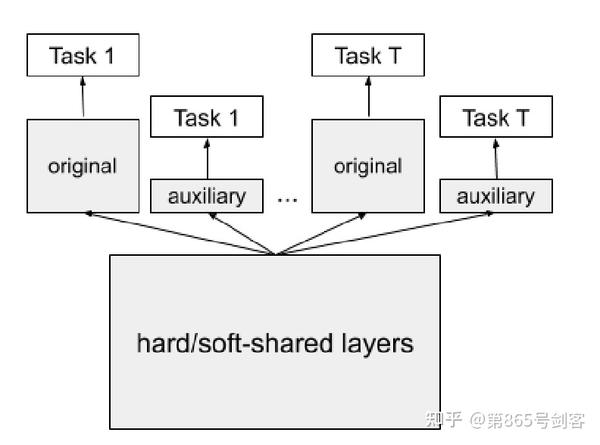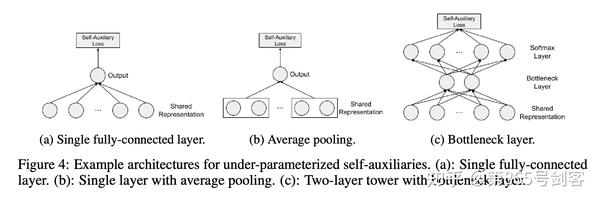1.我们都知道over-parameterization对单任务模型是有价值的，那边对多任务模型是否成立？

2.通过实验对比了大型模型和小型模型进行多目标学习中的不同表现。

To summarize our insights, for a multi-task learning model, small models benefit from good multi-task generalization but hurts Pareto efficiency; big models theoretically have better Pareto efficiency but could suffer from loss of generalization.

$$f_{t}(x; \theta_{sh}, \theta_{t})=f_{t}(h(x; \theta_{sh}); \theta_{t}), \forall t$$

$$f_t^a(x; \theta_{sh}, \theta_t^a))=f_t(h(x;\theta_{sh}); \theta_t^a), \forall t$$$$\hat{L}(\theta)=\sum_{t=1}^Tw_t(\hat{L}(\theta_{sh},\theta_t)+\gamma \hat{L}(\theta_{sh},\theta_t^a))$$

$$\hat{L}(\theta_{sh},\theta_t^a))=\frac{1}{n}\sum_{i=1}^nL_t(f_t^a(x; \theta_{sh}, \theta_t^a)), y_i^t)$$PS: Over-Parameterization的说明见Reconciling modern machine-learning practice and the classical bias–variance trade-off, Two models of double descent for weak features等文章,或者知乎相关讨论